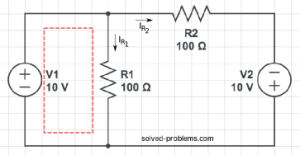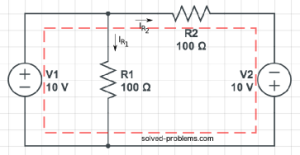Find resistor currents using KVL.Solution:andare parallel. So the voltage acrossis equal to. This can be also calculated using KVL in the left hand side loop:.
Now, use Ohm’s law to find:.
To find, write KVL around the outer loop:.
Again, use Ohm’s law to determine:.

Now, tell me what is the current passing through?

Hi! Yaz is here. I am passionate about learning and teaching. I try to explain every detail simultaneously with examples to ensure that students will remember them later too.

## Join the Conversation

1.2.3.4.5.6.1. 0.3

1. good job!
From KCL:2.yongule says:

good work i got aleast some concepts

1. good, very good. let me know if you need any help.

1.shahidul islam says:

My Circuit

1.andare both short circuited. If you check the circuit you can easily see that both terminals are connected to each other for both.
So, you have a circuit with asource andand Ohm’s law says:Let me know if you need more help.

1.shahidul islam says:

Thank you very much, sir.

Surprisingly, in every guidebook I found the answer 7.5 Amp where there was no explanation behind the answer. But now I know the explanation and also know that correct answer is 5 Amp.

I will post here if I need further help. Thanks again for your help.

2. You are welcome.

2.prasad says:

eliminate 10ohm resister becuase current will flow through low resistance patch then it is a series resistance circuit easy you can calculate otherwise let me know

3.Thomas says:

What happens if you reverse the polarity of V2?

1. good question.
Actually, it will be quite interesting. The voltage acrossisand therefore, the voltage drop onwill be zero. You can also verify this by KVL around the outer loop:And using the Ohm’s law, we conclude that no current passes through. The current ofis the same as before because its voltage is not changed.

4.Min Dhami says:

please give me solve two voltage source are given